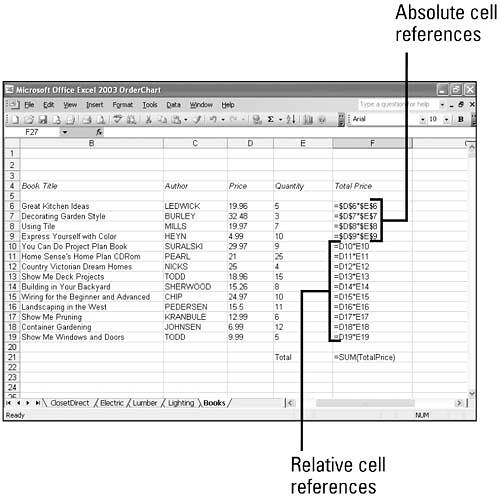# Understanding Cell Referencing

### Understanding Cell Referencing

Each cell, the intersection of a column and row on a worksheet, has a unique address, or cell reference , based on its column letter and row number. For example, the cell reference for the intersection of column D and row 4 is D4.

#### Cell References in Formulas

The simplest formula refers to a cell. If you want one cell to contain the same value as another cell, type an equal sign followed by the cell reference, such as =D4. The cell that contains the formula is known as a dependent cell because its value depends on the value in another cell. Whenever the cell that the formula refers to changes, the cell that contains the formula also changes.

Depending on your task, you can use either relative cell references, which are references to cells relative to the position of the formula, or absolute cell references, which are cell references that always refer to cells in a specific location.

#### Relative Cell References

When you copy and paste or move a formula that uses relative references, the references in the formula change to reflect cells that are in the same relative position to the formula. The formula is the same, but it uses the new cells in its calculation. Relative addressing eliminates the tedium of creating new formulas for each row or column in a worksheet filled with repetitive information.

#### Absolute Cell References

If you don't want a cell reference to change when you copy a formula, make it an absolute reference by typing a dollar sign (\$) before each part of the reference that you don't want to change. You can add a \$ before the column letter, the row number, or both. To ensure accuracy and simplify updates, enter constant values (such as tax rates, hourly rates, and so on) in a cell, and then use absolute references to them in formulas.Show Me Microsoft Office Excel 2003
ISBN: 0789730057
EAN: 2147483647
Year: 2002
Pages: 291